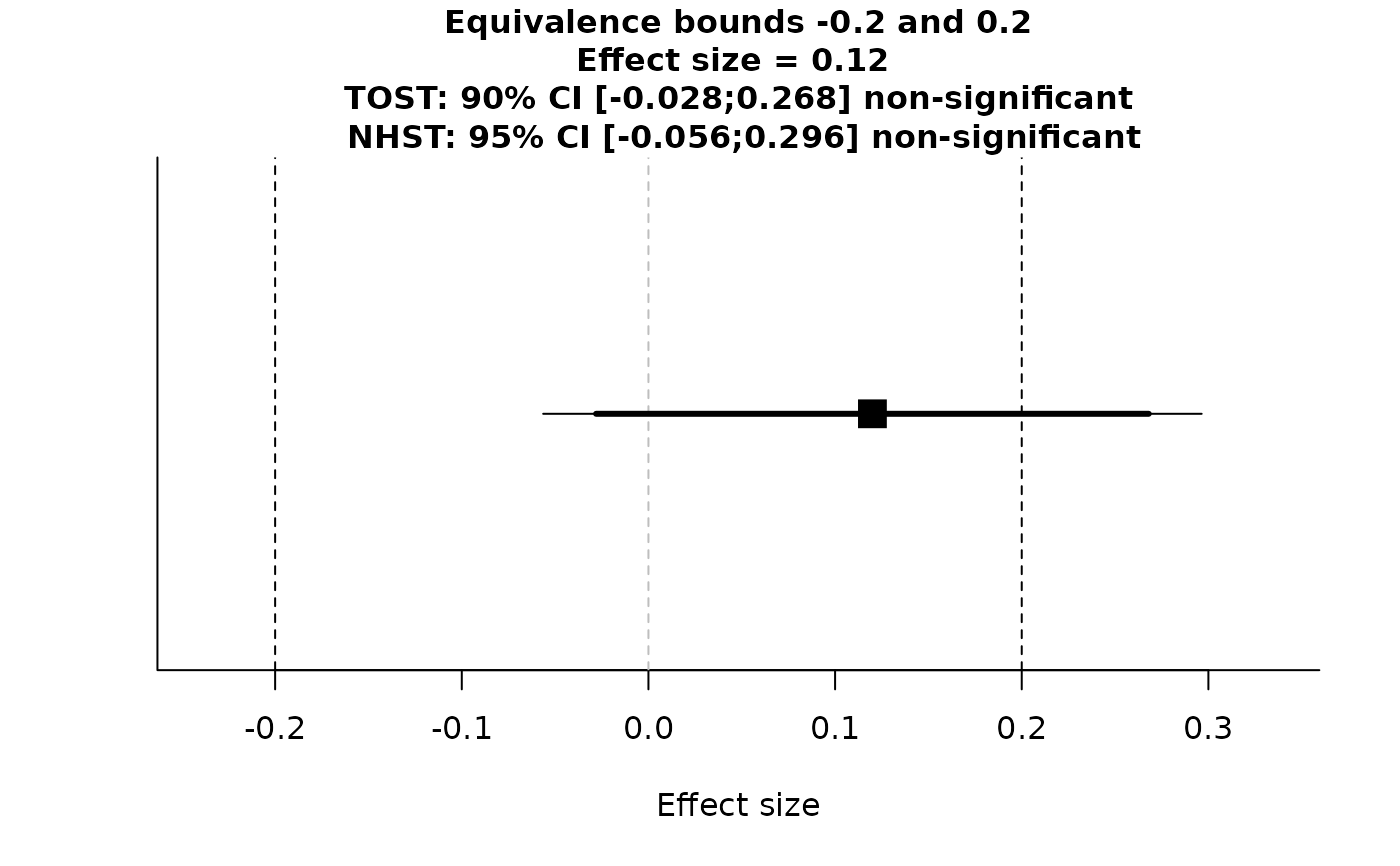TOST function for meta-analysis

TOSTmeta(
ES,
var,
se,
low_eqbound_d,
high_eqbound_d,
alpha,
plot = TRUE,
verbose = TRUE
)

## Arguments

ES

meta-analytic effect size

var

meta-analytic variance

se

standard error

low_eqbound_d

lower equivalence bounds (e.g., -0.5) expressed in standardized mean difference (Cohen's d)

high_eqbound_d

upper equivalence bounds (e.g., 0.5) expressed in standardized mean difference (Cohen's d)

alpha

alpha level (default = 0.05)

plot

set whether results should be plotted (plot = TRUE) or not (plot = FALSE) - defaults to TRUE

verbose

logical variable indicating whether text output should be generated (verbose = TRUE) or not (verbose = FALSE) - default to TRUE

## Value

Returns TOST Z-value 1, TOST p-value 1, TOST Z-value 2, TOST p-value 2, alpha, low equivalence bound d, high equivalence bound d, Lower limit confidence interval TOST, Upper limit confidence interval TOST

## Examples

## Run TOSTmeta by specifying the standard error
TOSTmeta(ES=0.12, se=0.09, low_eqbound_d=-0.2, high_eqbound_d=0.2, alpha=0.05)
#> TOST results:
#> Z-value lower bound: 3.56 	p-value lower bound: 0.0002
#> Z-value upper bound: -0.889 	p-value upper bound: 0.187
#>
#> Equivalence bounds (Cohen's d):
#> low eqbound: -0.2
#> high eqbound: 0.2
#>
#> TOST confidence interval:
#> lower bound 90% CI: -0.028
#> upper bound 90% CI:  0.268
#>
#> NHST confidence interval:
#> lower bound 95% CI: -0.056
#> upper bound 95% CI:  0.296
#>
#> Equivalence Test Result:
#> The equivalence test was non-significant, Z = -0.889, p = 0.187, given equivalence bounds of -0.200 and 0.200 and an alpha of 0.05.
#>
#>
#> Null Hypothesis Test Result:
#> The null hypothesis test was non-significant, Z = 1.333, p = 0.182, given an alpha of 0.05.
#>
#>
#> NHST: don't reject null significance hypothesis that the effect is equal to 0
#> TOST: don't reject null equivalence hypothesis
## Run TOSTmeta by specifying the variance
TOSTmeta(ES=0.12, var=0.0081, low_eqbound_d=-0.2, high_eqbound_d=0.2, alpha=0.05)
#> TOST results:
#> Z-value lower bound: 3.56 	p-value lower bound: 0.0002
#> Z-value upper bound: -0.889 	p-value upper bound: 0.187
#>
#> Equivalence bounds (Cohen's d):
#> low eqbound: -0.2
#> high eqbound: 0.2
#>
#> TOST confidence interval:
#> lower bound 90% CI: -0.028
#> upper bound 90% CI:  0.268
#>
#> NHST confidence interval:
#> lower bound 95% CI: -0.056
#> upper bound 95% CI:  0.296
#>
#> Equivalence Test Result:
#> The equivalence test was non-significant, Z = -0.889, p = 0.187, given equivalence bounds of -0.200 and 0.200 and an alpha of 0.05.
#>
#>
#> Null Hypothesis Test Result:
#> The null hypothesis test was non-significant, Z = 1.333, p = 0.182, given an alpha of 0.05.
#>
#>
#> NHST: don't reject null significance hypothesis that the effect is equal to 0
#> TOST: don't reject null equivalence hypothesis## If both variance and se are specified, TOSTmeta will use standard error and ignore variance
TOSTmeta(ES=0.12, var=9999, se = 0.09, low_eqbound_d=-0.2, high_eqbound_d=0.2, alpha=0.05)
#> TOST results:
#> Z-value lower bound: 3.56 	p-value lower bound: 0.0002
#> Z-value upper bound: -0.889 	p-value upper bound: 0.187
#>
#> Equivalence bounds (Cohen's d):
#> low eqbound: -0.2
#> high eqbound: 0.2
#>
#> TOST confidence interval:
#> lower bound 90% CI: -0.028
#> upper bound 90% CI:  0.268
#>
#> NHST confidence interval:
#> lower bound 95% CI: -0.056
#> upper bound 95% CI:  0.296
#>
#> Equivalence Test Result:
#> The equivalence test was non-significant, Z = -0.889, p = 0.187, given equivalence bounds of -0.200 and 0.200 and an alpha of 0.05.
#>
#>
#> Null Hypothesis Test Result:
#> The null hypothesis test was non-significant, Z = 1.333, p = 0.182, given an alpha of 0.05.
#>
#>
#> NHST: don't reject null significance hypothesis that the effect is equal to 0
#> TOST: don't reject null equivalence hypothesis